﻿ 广元市人民检察院·四川省青川县人民检察院公告       ·旺苍县人民检察院公告       ·中共四川省人民检察院党组巡察公告       ·欢迎关注中央政法委、省委政法委新媒体账号二维码       ·关于限期主动说清“一卡通”问题的通告利州 昭化 朝天 苍溪 剑阁 旺苍 青川 荣山本院动态

• []
• []
• []
• []
• []
• []
• []
• []
• []
• []本院概况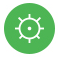机构职能班子成员公开规定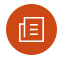检察常识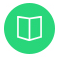专项整治12309检察服务中心起诉书查询

• []
• []
• []
• []
• []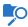案件动态

• []
• []
• []
• []
• []
• []
• []
• []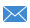检察长信箱

 信件编号 信件密码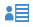律师预约

 信件编号 信件密码诉讼进度及结果查询

2019-09-10

2019-09-10检察新闻

• []
• []
• []
• []
• []
• []以案说法

• []
• []
• []
• []
• []
• []队伍建设

• []
• []
• []
• []
• []
• []
• []
• []基层检察

• []
• []
• []
• []
• []
• []
• []
• []
 名次 单位 发文总数 1 剑阁县院 110 2 苍溪县院 81 3 利州区院 80 4 昭化区院 79 5 朝天区院 61 6 旺苍县院 43 7 青川县院 31理论研究

• []
• []
• []
• []检察文化新媒体矩阵COPYRIGHT 2019 广元市人民检察院 ALLRIGHT RESERVED技术支持：程友科技微信公众号(scgyjcy01) 警示教育基地安卓端